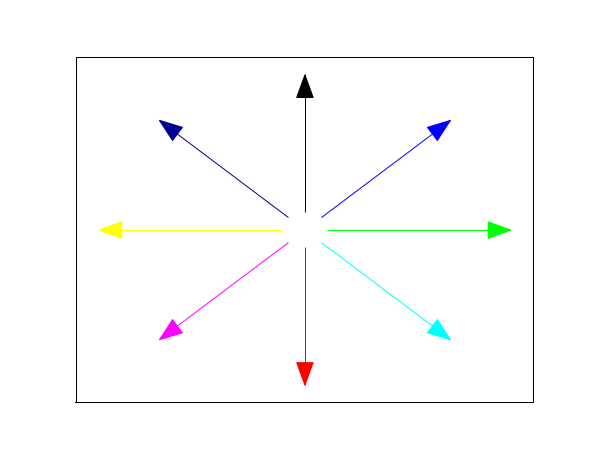Scilab Home page | Wiki | Bug tracker | Forge | Mailing list archives | ATOMS | File exchange
Change language to: Français - Português - 日本語 - Русский

Please note that the recommended version of Scilab is 6.1.1. This page might be outdated.
See the recommended documentation of this function

Scilab help >> Graphics > geometric_shapes > xarrows

# xarrows

draw a set of arrows

### Calling Sequence

`xarrows(nx, ny, [arsize, color])`

### Arguments

nx, ny

real vectors or matrices of same size.

arsize

a real scalar, size of the arrow head. The default value can be obtained by setting `arsize` to `-1`.

color

a matrix or scalar. If `color` is a positive scalar it gives the color to use for all arrows. If it is a negative scalar then the current color is used. If it is a vector `color(i)` gives the color to use for arrow `i`.

### Description

`xarrows` draws a set of arrows given by `nx` and `ny`. If `nx` and `ny` are vectors, the `i`-th arrow is defined by `(nx(i),ny(i))-->(nx(i+1),ny(i+1))`. If `nx` and `ny` are matrices:

```nx=[xi_1 x1_2 ...; xf_1 xf_2 ...]
ny=[yi_1 y1_2 ...; yf_1 yf_2 ...]```

the `k`-th arrow is defined by `(xi_k,yi_k)-->(xf_k,yf_k)`.

`xarrows` uses the current graphics scale which can be set by calling a high level drawing function such as `plot2d`.

### Examples

```x = 2*%pi*(0:7)/8
x1 = [sin(x); 9*sin(x)];
y1 = [cos(x); 9*cos(x)];
plot2d([-10,10], [-10,10], [-1,-1], "022")
xset("clipgrf")
xarrows(x1, y1, -1, [1:7,9]) //8-th color is white
xset("clipoff")```